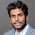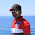Sun Saathiya (ABCD 2) Piano Notes Full Song

Sun saathiya maahiya
A -E -D E +A A E D E +  A -G
Barsa de ishqa ki syahiyaan

D E D E D E + A A E D E +A A

Rang jaaun, rang rang jaaun ri, haari main
E D E D E D E D E D C B  C +E -B C B A
Tujhpe main jhar jhar jhar jaaun

E D E D E D E D E D C B  C +E -B C B A
Hoon piya bas teri main

E E E+ C -B A G F E D C
Ho chhu le toh khari main toh khari main khari main

A G F E E E +C -B A G G G G G +B B +C E
Sun saathiya maahiya
A -E -D E +A A E D E +  A -G
Barsa de ishqa ki syahiyaan

D E D E D E + A A E D E +A A

Main ret si, boond ka zariya tu
E F D E F D  E D -C B C B A
Paa ke tujhe bheeg jaaun re

E F D E  +A  B C B A F E.......X2

Rang jaaun, rang rang jaaun ri, haari main
E D E D E D E D E D C B  C +E -B C B A
Tujhpe main jhar jhar jhar jaaun

E D E D E D E D E D C B  C +E -B C B A
Hoon piya bas teri main

E E E+ C -B A G F E D C
Ho chhu le toh khari main toh khari main khari main

A G F E E E +C -B A G G G G G +B B +C E

Sun saathiya maahiya
A -E -D E +A A E D E +  A -G
Barsa de ishqa ki syahiyaan

D E D E D E + A A E D E +A A

Related Posts

Previous
Next Post »